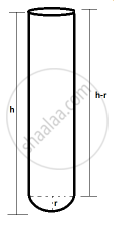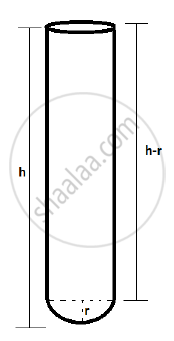SSC (Marathi Semi-English) 10thMaharashtra State Board
Share

# A Test Tube Has Diameter 20 Mm and Height is 15 Cm. the Lower Portion is a Hemisphere. Find the Capacity of the Test Tube - SSC (Marathi Semi-English) 10th - Geometry

ConceptSurface Area of a Combination of Solids

#### Question

A test tube has diameter 20 mm and height is 15 cm. The lower portion is a hemisphere. Find the capacity of the test tube. (π = 3.14)#### SolutionRadius of test tube (r) =20/2= 10 mm = 1 cm

Height of test tube = 15 cm

Upper portion of test tube is cylinder and lower portion of test tube is hemisphere.

Height of cylinder (H)= h-r = 15 - 1 = 14cm

Volume of test tube = Volume of cylinder + Volume of hemisphere

= pir^2H+2/3pir^3

= (3.14)(1)^2(14)+2/3(3.14)(1)^3

= 43.96 + 2.09

= 46.05 cm3

Capacity of test tube is 46.05 cm3.

Is there an error in this question or solution?

#### APPEARS IN

2014-2015 (March) (with solutions)
Question 4.3 | 4.00 marks
Solution A Test Tube Has Diameter 20 Mm and Height is 15 Cm. the Lower Portion is a Hemisphere. Find the Capacity of the Test Tube Concept: Surface Area of a Combination of Solids.
S